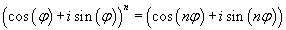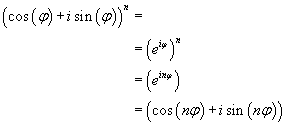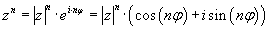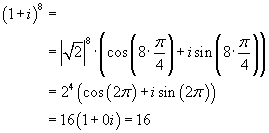# Can you prove DeMoivre's theorem

The sentence by Moivre Andreas Pester University of Applied Sciences K rnten, Villach
[email protected] Summary: Brief derivation of Moivre's theorem and its application to the exponentiation of complex numbers. Main pageKeywords: Moivre's theorem | Raising complex numbers to the power of | The complex power function | Equation 1 | Equation 2 | Example 1 | Example 2 The following theorem for integer exponents n follows from Euler's formula according to the laws of power calculation:because it appliesIf one applies theorem (1) to any complex number z = |z| · Ei · f on, that's how you get the formulafor raising complex numbers to the power. Example 1:One would also have the example using the binomial formula for (a + b)n can solve, but with increasing power and for non-integer real and imaginary parts, the numerical effort becomes relatively high. Note: There cos and sin are periodic functions with the smallest period 2p and an integral multiple of 2p also in turn period of cos and sin is, the result of raising a complex number to the power of an integer exponent is uniquely determined. However, this cannot be transferred to rational, real or complex exponents. For this see the square root of complex numbers and the complex power function. Now that it is clear what the power of a complex number means and how it can be calculated, one can go a step further and use the complex power function f (z) = ez introduce. ez = e(Re(z) + i Im (z)) = e(Re(z)· Ei Im (z)

Otherwise the laws of power calculus apply, which are transferred.

Example 2:
e(2 + i p / 2) = e2· Ei p / 2 = e2· I